# Stochastic Galerkin with low-rank tensor representation¶

Recall our parametrized differential equation with solution $u\in V=L^2(\Gamma, \mu; H_0^1(D))$

\begin{align} \begin{array}{rclll} -\operatorname{div}\kappa(x, y)\nabla u(x, y) & = & f(x)& \text{in } D\times\Gamma \\ u(x, y) & = & 0 & \text{on } \partial D\times \Gamma. \end{array} \end{align}

As in the previous section, we need to restrict the approximation space $V$ to something finite dimensional. Here, we employ the truncation $M\in\mathbb{N}$ and FE approximation space $X_h$ as before. For the restriction of the generalized polynomial chaos approximation, we consider the full tensor set of order $M$ and degree $\boldsymbol d=(d_1, \ldots, d_M)$

\begin{align} \Lambda := \{\alpha=(\alpha_1, \ldots, \alpha_M, 0, \ldots )\in\mathbb{N}^\infty\;\vert\; \alpha_m=1,\ldots, d_m, m=1,\ldots, M\}. \end{align}

Then, the discrete space $S_{\Lambda}^M:= \mathrm{span}\{P_\alpha, \alpha\in\Lambda\}$, together with $X_h=\mathrm{span}\{\phi_i, i=1,\ldots, N\}$ we obtain the approximation space $V_{h, \Lambda}^M = S_{\Lambda}^M \otimes X_h$. Every element $u\in V_{h, \Lambda}^M$ can be written in basis representation

\begin{align} u(x, y) = \sum_{i=1}^N\sum_{\alpha\in\Lambda} U(i, \alpha)\phi_i(x)P_\alpha(y). \end{align}

The tensor $U\in\mathbb{R}^{N, d_1,\ldots, d_M}$ suffers from the curse of dimensionality and its low-rank reduction is our main goal in this section.

The diffusion coefficient in this section is considered to be a random field $\kappa\in L^\infty(\Gamma^M, \mu^M; L^\infty(D))$ that is represented by a polynomial chaos basis in $S_\Lambda^M$ and FE functions in $X_h$. As before, $\Gamma^M$ and $\mu^M$ denote the tensorization of the one-dimensional parameter domain $\Gamma^M = \times_{m=1}^M\Gamma^m$ and measure $\mu^M=\otimes_{m=1}^M\mu^m$, respectively. Moreover, a low-rank assumption is employed, in the sense that there exists a TT-rank $\boldsymbol r=(r_0,\ldots, r_{M-1})$ such that

\begin{align} \tag{exTT} \kappa(x, y) = \sum_{k_0=1}^{r_0}\dots\sum_{k_{M-1}=1}^{r_{M-1}} \sum_{i=1}^N A_0(i, k_0)\phi_i(x)\prod_{m=1}^M \sum_{\alpha_m=1}^{d_m}A_m(k_{m-1}, \alpha_m, k_m)P_{\alpha_m}(y_m), \qquad k_M=1 \text{ is neglected} \end{align}

This representation is assumed to be exact, otherwise, we need to be more careful in the definition of the bilinear form and the interpretation of the solution.

### Example I: affine diffusion¶

The fact that this format is achieved in practical applications can be seen when considering the affine coefficient \begin{align} \tag{1} \kappa(x, y) = \kappa_0(x) + \sum_{m=1}^M \kappa_m(x) y_m. \end{align} This function can be written as the matrix-vector multiplication

\begin{align} \kappa(x, y) = \underbrace{\begin{bmatrix} \kappa_0(x)&\cdots&\kappa_{M}(x) \end{bmatrix}}_{[A_0(x, k_0)]} \underbrace{\begin{bmatrix} 1 & 0 & 0 & \cdots & 0 \\ 0 & y_1 & 0 & \cdots & 0 \\ 0 & 0 & 1 & \cdots & 0 \\ \vdots & & & \ddots & \vdots \\ 0 & & \cdots & & 1 \\ \end{bmatrix}}_{[A_1(k_0, y_1, k_1]} \underbrace{\begin{bmatrix} 1 & 0 & 0 & \cdots & 0 \\ 0 & 1 & 0 & \cdots & 0 \\ 0 & 0 & y_2 & \cdots & 0 \\ \vdots & & & \ddots & \vdots \\ 0 & & \cdots & & 1 \\ \end{bmatrix}}_{[A_2(k_1, y_2, k_2)]} \cdots \underbrace{\begin{bmatrix} 1 & 0 & 0 & \cdots & 0 \\ 0 & 1 & 0 & \cdots & 0 \\ 0 & 0 & 1 & \cdots & 0 \\ \vdots & & & \ddots & \vdots \\ 0 & & \cdots & & y_M \\ \end{bmatrix} \begin{bmatrix} 1\\ \\ \vdots \\ \\ 1 \end{bmatrix}}_{[A_{M}(k_{M-1}, y_M)]} \end{align}

which can be interpreted as rank $\boldsymbol r=(M+1, \ldots, M+1)$ tensor train. This observation is obvious, since the function (1) can be seen as a rank $M+1$ canonical tensor, which has a tensor train representation of rank $\boldsymbol r$.

### Example II: Reconstruction or HOSVD¶

As in the lecture, every high-dimensional tensor can be represented as tensor-train, when applying the higher order singular value decomposition, without truncation. An approximation in a possible low-rank tensor, is obtained when singular value thresholding is considered. As an alternative, the tensor train reconstruction algorithm can be used. This method rely on samples of a function $\kappa\colon \Gamma\to X_h\subset X$ and creates a tensor train that minimizes the empirical functional \begin{align} \mathcal{J}(\Phi) = \frac{1}{N}\sum_{i=1}^{N_s}\|\Phi(y_i) - \kappa(y_i)\|_{X} \end{align} over the set of all functions having a representation as in (exTT). This procedure is known as variational Monte-Carlo method.

#### Exercise: Tensor Reconstruction using Xerus¶

A simple way to make use of the tensor train reconstruction is to employ the functionality in Xerus. We want to find a tensor train that represents the affine coefficient field from the previous examples.

In :
from __future__ import division
import numpy as np
from dolfin import *
import matplotlib as mpl
import matplotlib.pyplot as plt
import scipy.sparse as sps
import xerus as xe
from coef_field import CoefField               # CoefField of tutorial 1
parameters.linear_algebra_backend = 'Eigen'    # fenics internal matrix system
%matplotlib inline

M = 5                                          # number of dimensions in coef expansion
mean = 1.0                                     # mean value of affine field
decay = 2.0                                    # decay parameter of eigenfunctions
scale = 1.0                                    # multiplicative scale
field = CoefField(M=M, mean=mean, decay=decay, scale=scale)
mesh = UnitSquareMesh(10, 10)                  # FEniCS mesh on a square
fs = FunctionSpace(mesh, 'CG', 1)              # FunctionSpace definition


We create a set of $N_s\in\mathbb{N}$ uniform samples in $(-1, 1)^M$ and compute the corresponding field realisations.

In :
Ns = 100
samples = []
measurements = []
for lia in range(Ns):
y = np.random.rand(M)*2 - 1
value = field.realisation(y, fs).vector().array()
samples.append(y)
measurements.append(value)


The obtained samples and measurements are given to a xerus measurement set and the reconstruction is started.

In :
poly_dim = 5                                 # desired polynomial degree
adf_tol = 1e-8                               # solver tolerance
adf_iter = 10000                             # maximal number of iteration
xe_measurements = xe.UQMeasurementSet()      # create Xerus measurement Set
for y, value in zip(samples, measurements):
xe_measurements.add(y, xe.Tensor.from_ndarray(value))
basis = xe.PolynomBasis.Legendre             # define flag of used polynomials in xerus
dimensions = [fs.dim()] + [poly_dim] * M     # set dimensions
res = xe.uq_ra_adf(xe_measurements, basis, dimensions, adf_tol, adf_iter)
print(res)

<xerus.TTTensor object at 0x7f86e0c44cd0>


Having a look at the terminal output of the notebook, we observe that the error on a Controlset is approximately $10^{-9}$. Some properties of the resulting tensor train are given here:

In :
print("TT-dimension: {}".format(res.dimensions))
print("finite element # basis function: {}".format(fs.dim()))
print("TT-rank: {}".format(res.ranks()))

TT-dimension: [121, 5, 5, 5, 5, 5]
finite element # basis function: 121
TT-rank: [6, 5, 4, 3, 2]


For the evaluation of the tensor train in the format as in (exTT) we need a method that takes a sample $y_i$ and returns the corresponding finite element function. Having the polynomial evaluation at hand, we only need to compute the matrix vector product of the tensor components.

In :
import numpy.polynomial.legendre as leg        # polynomial evaluation and quadrature
def polyval(x, nu, normalised=False):
# the polynomials given by leg.legval are not normalized
if normalised:
fn = np.sqrt((2*nu+1))
else:
fn = 1
return leg.legval(x, *nu+)*fn

def set_fem_fun(vec, fs):
_retval = Function(fs)
_retval.vector().set_local(vec)
return _retval

def eval_ex_tt(comps, y, fs, normalised=False):
assert len(y) == len(comps)-1
retval = 1
for lia in range(len(comps)-1, 0, -1):
comp = comps[lia]
values = np.zeros(comp.shape)
for lib in range(comp.shape):
values[lib] = polyval(y[lia-1], lib, normalised=normalised)
if lia == len(comps) - 1:
retval = np.dot(comp[:, :, 0], values)
continue
_comp = comp.transpose(0, 2, 1)         # else, transpose [k_m, n, k_m+1] -> [k_m, k_m+1, n]
#                                       # reshape -> [k_m*k_m+1, n]
_comp = _comp.reshape((comp.shape * comp.shape, comp.shape), order="F")
_comp = _comp.dot(values)               # dot -> [k_m*k_m+1]
#                                       # reshape -> [k_m, k_m+1]
_comp = _comp.reshape((comp.shape, comp.shape), order="F")
retval = _comp.dot(retval)              # dot -> [k_m]
retval = comps[0, :, :].dot(retval)
return set_fem_fun(retval, fs)


We test the result on some samples and show the true coefficient realisations along with the result of the tensor approximation and the absolute difference.

In :
# Store component tensors of the xerus tensor in a list
comps = [xe.Tensor.to_ndarray(res.get_component(lia)) for lia in range(len(res.dimensions))]
mpl.rcParams['figure.figsize'] = [10, 3]
for lia in range(3):
y = np.random.rand(M)*2 -1
truth = field.realisation(y, fs)
approx = eval_ex_tt(comps, y, fs)
diff = set_fem_fun(np.abs(truth.vector().array() - approx.vector().array()), fs)
plt.figure()
plt.subplot(131)
plt.title("True sample")
im = plot(truth)
plt.colorbar(im)
plt.subplot(132)
plt.title("TT approximation")
im = plot(approx)
plt.colorbar(im)
plt.subplot(133)
im = plot(diff)
plt.title("Difference")
plt.colorbar(im)
plt.tight_layout()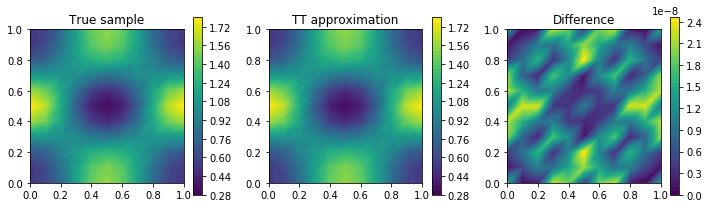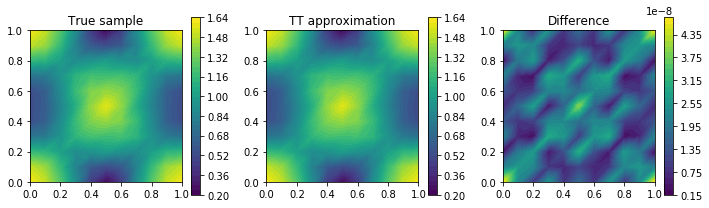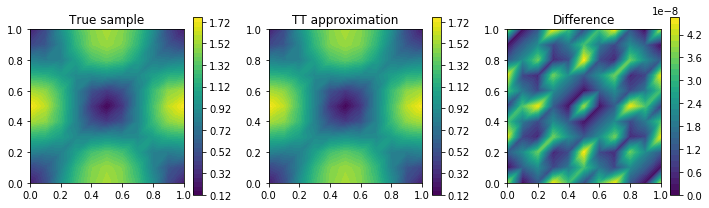The resulting tensor approximation is of high order and very efficiently computed with only $N_s=100$ samples. This is due to the fact, that the affine coefficient is holomorphic and linear in $y$, hence it can be approximated with polynomials with high accuracy. Furthermore, the rank of the tensor approximation is known a priori as maximal $M+1$, which is attained, as one can see above. The decreasing ranks for higher modes $m=2, 3, 4, 5\;\; (r_m=5, 4, 3, 2)$ is explained by the employed eigenfunction decay $\sigma=2$.

### Galerkin approximation with tensor train coefficient¶

We continue with the formulation of the Galerkin approximation of the model problem, having a diffusion coefficient $\kappa^{\mathrm{TT}}$, given as tensor train (exTT). The discrete problem reads:

Find $u_{h} \in V_{h, \Lambda}^M$ such that for all $v_h\in V_{h, \Lambda}^M$

\begin{align} a(u_h, v_h) = \int\limits_{\Gamma^M}\int\limits_{D} \kappa^{\mathrm{TT}}(x,y)\nabla u_h(x,y)\cdot \nabla v_h(x,y)\ \mathrm{d}x\ \mathrm{d}\mu^M(y) &=\int\limits_{\Gamma^M}\int\limits_{D} f(x) v_h(x,y)\ \mathrm{d}x\ \mathrm{d}\mu^M(y) = \ell(v_h). \end{align}

Employing the tensor format and change the ansatz and test function to basis elements of $V_{h, \Lambda}^M$ one obtains the discrete bilinear form for every $i, i'=1, \ldots, N$ and multi-index $\alpha, \alpha'i\in\Lambda$

\begin{align} a(i, i', \alpha, \alpha') := \sum_{k_0=1}^{r_0}\dots \sum_{k_{M-1}=1}^{r_{M-1}} \sum_{j=1}^{N}A_0(j, k_0) \int_D \phi_j \nabla\phi_i\cdot\nabla\phi_{i'}\mathrm{d}x \prod_{m=1}^M \sum_{\nu_m=1}^{d_m}A_m(k_{m-1}, \nu_m, k_m) \int_{\Gamma^m} P_{\nu_m}P_{\alpha_m} P_{\alpha_m'}\mathrm{d}\gamma^m. \end{align}

This formula shows, the choice of basis representation for the diffusion coefficient is not limit to the one that defines $V_{h, \Lambda}^M$. As in deterministic finite element theory, it is sufficient to choose a discontinuous basis on $D$ with polynomial degree of lower order. The same holds in the stochastic space, where a sufficient basis can be chosen.

For the right-hand side, due to its deterministic character, we obtain \begin{align} \ell(i, \alpha) = \int_D f(x)\phi_{i}(x)\mathrm{d}x\prod_{m=1}^M\int_{\Gamma^m}P_{\alpha_m}(y_m)\mathrm{d}\gamma^m, \end{align} where the stochastic integrals are trivial, since the polynomials are chosen orthonormal $P_0(y_m) = 1$.

Finally, the linear system of equations that needs to be solved states: find $U\in\mathbb{R}^{N, d_1,\ldots, d_M}$, such that for every $i=1,\ldots, N$ and $\alpha\in\Lambda$ \begin{align} \tag{LES} \sum_{\alpha'\in\Lambda}\sum_{i'=1}^N a(i, i', \alpha, \alpha') U(i', \alpha') = \ell(i, \alpha). \end{align}

#### Exercise stochastic Galerkin with a tensor train coefficient¶

Implement the stochastic Galerkin system for the diffusion problem with a coefficient, compressed as low-rank tensor train. Solve the algebraic linear system using the Xerus implementation of the Alternating Least Squares (ALS) algorithm.

In :
assert comps is not None                  # reuse the reconstructed coefficient above
coef_comps = comps
poly_degs = *M
sol_ranks =  + *M + 
coef_ranks =  + res.ranks() + 

rhs = Constant(1.0)
# rhs = Expression("sin(2*pi*x)*cos(2*pi*x) + x*x", degree=5)
rhs = project(rhs, fs)

# Define boundary condition
u0 = Constant(0.0)
bc = DirichletBC(fs, u0, 'on_boundary')
FEM = {"fs": fs,
"bc": bc}


The following method implements the physical component of the discrete bilinear form and linear form. More precisely, for every $k_0=1,\ldots, r_0$ and $i, i'=1,\ldots, N$, one stores \begin{align} a_0(i, i', k_0) = \sum_{j=1}^N A_0(j, k_0) \int_D \phi_i \nabla\phi_i\cdot \nabla\phi_{i'}\mathrm{d}x \end{align} and \begin{align} \ell_0(i, 1) = \int_Df(x)\phi_i(x)\mathrm{d}x. \end{align}

In :
def compute_fem_comp(coef, rhs, FEM):
assert isinstance(rhs, Function)
fs = FEM["fs"]
bc = FEM["bc"]
u, v = TrialFunction(fs), TestFunction(fs)

dof2val = bc.get_boundary_values()
bc_dofs = dof2val.keys()

# setup rhs component
B = []
B_buf = assemble(rhs * v * dx)
bc.apply(B_buf)
B.append(B_buf.array())
B_0 = np.array(B).T
# setup coef components
A_0 = []
for k in range(coef.shape):
coef_fun = set_fem_fun(coef[:, k], fs)
_A = assemble(coef_fun * inner(nabla_grad(u), nabla_grad(v)) * dx)
bc.zero(_A)
rows, cols, values = as_backend_type(_A).data()
A_0.append(sps.csr_matrix((values, cols, rows)))
return A_0, B_0, bc_dofs


Next, we need to take care of the stochastic components. Hence, the tensor components of the bilinear and linear form are constructed for every dimension $m=1,\ldots, M$, multi-index $\alpha, \alpha'\in\Lambda$ and rank $k_{m-1}=1,\ldots, r_{m-1}$ and $k_m=1,\ldots, r_m$ as

\begin{align} a_m(k_{m-1}, \alpha_m, \alpha_m', k_m) = \sum_{\nu_m=1}^{d_m}A_m(k_{m-1}, \alpha_m, \alpha_m', k_{m})\int_{\Gamma^m} P_{\nu_m}P_{\alpha_m}P_{\alpha'_m}\mathrm{d}\gamma^m \end{align} and similar \begin{align} \ell_m(1, \alpha_m, 1) = \int_{\Gamma^m}P_{\alpha_m}\mathrm{d}\gamma^m = \delta_{1, \alpha_m}. \end{align}

In :
def generate_operator_tt(coef, ranks, degs):
cache_dict = {}                         # use a caching dictionary to not recompute the triple product
def tri_prod_int(mu, nu1, nu2):
if (mu, nu1, nu2) not in cache_dict:
nodes, weights = leg.leggauss(mu + nu1 + nu2 + 1)
# 0.5 for density
# polyval for coefficient (unnormalised Legendre polynomials)
# polyval for ansatz and test functions (normalised Leg. poly)
val = 0.5 * np.sum([w * polyval(n, mu) *
polyval(n, nu1, normalised=True) *
polyval(n, nu2, normalised=True)
for n, w in zip(nodes, weights)], axis=0)
cache_dict[(mu, nu1, nu2)] = val
return cache_dict[(mu, nu1, nu2)]

bf_cores = [None]
for i in range(len(coef)):
# init coef core (r_{m-1}, d_m, d_m, r_m)
opcorei = np.zeros([ranks[i+1],degs[i],degs[i],ranks[i+2]])
for nu1 in range(degs[i]):
for nu2 in range(degs[i]):
opcorei[:, nu1, nu2, :] = sum([coef[i][:,mu,:]*tri_prod_int(mu, nu1, nu2)
for mu in range(coef[i].shape)])

bf_cores.append(opcorei)

lf_cores = [None]
for i in range(len(coef)):
# rhs components are all equal e_1 = [1,0,...,0]
curr_rhs_comp = np.reshape(np.eye(degs[i], 1),
[1, degs[i], 1], order='F')
lf_cores.append(curr_rhs_comp)
return bf_cores, lf_cores


To collect the resulting tensor components, we call the written methods and assemble the bilinear and linear form. Note that the bilinear form consists of

• a list of $r_0$ sparse matrices: $[a_0(i, i', k_0)] \in \mathbb{R}^{N, N, r_0}$
• a list of $m=1, \ldots, M$ order 4 component tensors $[a_m(k_{m-1}, \alpha_m, \alpha_m, k_m)]\in\mathbb{R}^{r_{m-1}, d_m, d_m, r_m}$.

And the linear form is represented similarly using

• an assembled linear form $\ell_0\in\mathbb{R}^{1, N, 1}$
• a list of $m=1,\ldots, M$ order 3 component tensors $\ell_m\in\mathbb{R}^{1, d_m, 1}$ with $\ell_m(1, \alpha_m, 1) = \delta_{1, \alpha_m}$.
In :
# generate physical integral parts
A_0, B_0, bc_dofs = compute_fem_comp(coef_comps[0, :, :], rhs, FEM)
# generate stochastic integral parts
bf_cores, lf_cores = generate_operator_tt(coef_comps[1:], coef_ranks, poly_degs)

# add first physical component to bilinear form
bf_cores = []
for a_0 in A_0:
bf_cores.append(a_0)

# add first physical component to linear form
lf_cores = np.reshape(B_0, (1, B_0.shape, B_0.shape))


The incorporation of the homogenous boundary condition is left. Remember, in the definition of the physical components of the bilinear form, we deleted the rows that correspond to a boundary value, using bc.zero(_A). Hence, we need to set the diagonal entrys of those components to one. This is done by a boundary operator, that is constant in the stochastic components and either zero or one on the diagonal entry corresponding to a (non-) boundary coordinate. Afterwards, this operator is added to the original bilinear form operator.

The procedure of summing tensor operators, having sparse and non-sparse components is implemented in Xerus. We use a similar approach in a self written class called smatrix. The usage here is to reduce the amount of Code overflow.

In :
from tt_sparse_matrix import smatrix

A = smatrix(bf_cores)

# create sparse boundary operator
bcsparse_direct = sps.csr_matrix(A_0)
bcsparse_direct.data = np.zeros(len(bcsparse_direct.data))
bcsparse_direct[bc_dofs, bc_dofs] = 1
# create tensorized boundary operator
bcopcores = (M+1) * [[]]
bcopcores = [bcsparse_direct]
for i in range(M):
bcopcores[i + 1] = np.reshape(np.eye(bf_cores[i + 1].shape, bf_cores[i + 1].shape),
[1, bf_cores[i + 1].shape, bf_cores[i + 1].shape, 1], order='F')

BC = smatrix(bcopcores)
tt_bf = A + BC
tt_lf = lf_cores


Finally, the system of equations needs to be solved. We want to showcase the use of an implemented solver in Xerus. Since, we do not want to solve the system (LES) directly for it is of high-dimensionality, we employ a minimisation approach. This means, instead of solving (LES), we look for a tensor train $U\in\mathbb{R}^{N, d_1,\ldots, d_M}$ with

\begin{align} U = \mathrm{argmin} \|\mathcal{A}U-\mathcal{F} \|^2_F, \end{align}

where $\mathcal{A}$ and $\mathcal{F}$ are the computed tensor train bilinear and linear form.

The method of choice is an alternating least squares (ALS) method.

To do so, we first need to translate our objects into xerus TTOperator and TTTensor intances and define the solver.

In :
xe_op = tt_bf.to_xerus_op()         # cast smatrix operator to xerus operator using sparsity
# cast rhs to xerus tensor train
xe_rhs = xe.TTTensor([fs.dim()] + poly_degs)
for lia in range(M+1):
xe_rhs.set_component(lia, xe.Tensor.from_ndarray(tt_lf[lia]))
xe_rhs.canonicalize_left()
# define solver ALS method
als_solver = xe.ALSVariant(1, 0, xe.ALSVariant.lapack_solver, True)
als_solver.convergenceEpsilon = 1e-8
als_solver.useResidualForEndCriterion = True

pd = xe.PerformanceData(True)          # activates information printing

xe_sol = xe.TTTensor.random(xe_rhs.dimensions, sol_ranks[1:-1])
als_solver(xe_op, xe_sol, xe_rhs, pd)

print("Solution computed!")
print("TT-dimension: {}".format(xe_sol.dimensions))
print("finite element # basis function: {}".format(fs.dim()))
print("TT-rank: {}".format(xe_sol.ranks()))

Solution computed!
TT-dimension: [121, 4, 4, 4, 4, 4]
finite element # basis function: 121
TT-rank: [5, 5, 5, 5, 4]


The final result is an approximation to the original problem in low-rank tensor train format on the discrete space $V_{h, \Lambda}^M$, namely \begin{align} u(x, y) \approx u^{TT}(x, y) = \sum_{\alpha\in\Lambda}\sum_{i=1}^N U(i, \alpha)\phi_i(x)P_{\alpha}(y). \end{align} And written as tensor train \begin{align} u^{TT}(x,y) = \sum_{k_0=1}^{r_0}\dots \sum_{k_{M-1}=1}^{r_{M-1}}\sum_{i=1}^N U_0(i, k_0) \phi_i(x)\prod_{m=1}^M \sum_{\alpha_m=1}^{d_m}U_m(k_{m-1}, \alpha_m, k_m)P_{\alpha_m}(y_m). \end{align}

Let us compare the result. As for the coefficient field, we consider samples of the solution. On the one hand, as if we would have solved the partial differential equation with the specific field realisation, and on the other hand, as an evaluation of the tensor train result.

In :
def pw_solver(y):
u, v = TrialFunction(fs), TestFunction(fs)
_coef = field.realisation(y, fs)
_L = _coef * inner(nabla_grad(u), nabla_grad(v))*dx(fs.mesh())
_f = rhs * v * dx(fs.mesh())
_u = Function(fs)
solve(_L == _f, _u, bc)
return _u

In :
sol_comps = [xe.Tensor.to_ndarray(xe_sol.get_component(lia)) for lia in range(len(xe_sol.dimensions))]
mpl.rcParams['figure.figsize'] = [12, 3]
for lia in range(3):
y = np.random.rand(M)*2 -1
true_coef = field.realisation(y, fs)
truth = pw_solver(y)
approx = eval_ex_tt(sol_comps, y, fs, normalised=True)
diff = set_fem_fun(np.abs(truth.vector().array() - approx.vector().array())/np.linalg.norm(truth.vector().array()), fs)
plt.figure()
plt.subplot(141)
plt.title("True Coef")
im = plot(true_coef)
plt.colorbar(im)
plt.subplot(142)
plt.title("True sample")
im = plot(truth)
plt.colorbar(im)
plt.subplot(143)
plt.title("TT approximation")
im = plot(approx)
plt.colorbar(im)
plt.subplot(144)
im = plot(diff)
plt.title("Difference")
plt.colorbar(im)
plt.tight_layout()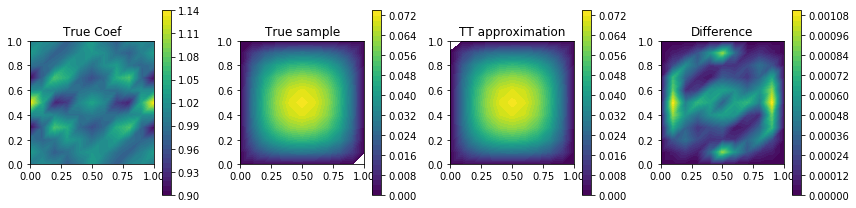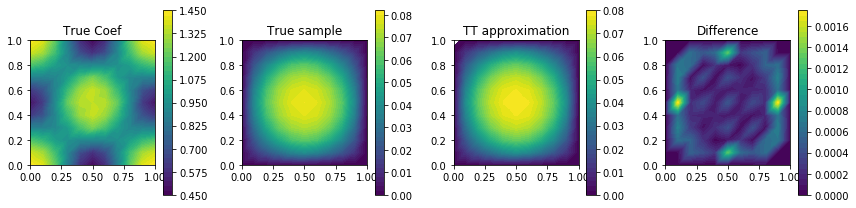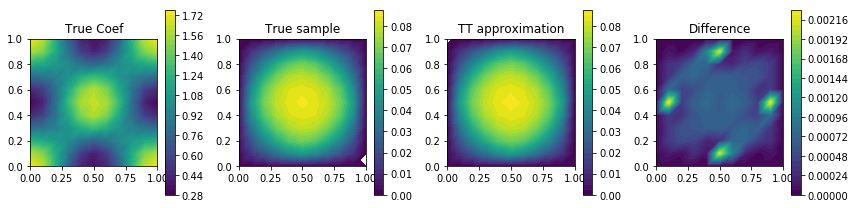To show off, let us demonstrate the importance of a surrogate by making a timing test. Therefore, we aim at the creation of 1000 samples for the solution, once for the true solution, and once for the surrogate evaluation.

In :
import time
N = 1000
y_list = []
for lia in range(N):
y_list.append(np.random.rand(M)*2 - 1)

mean_true = np.zeros(fs.dim())
start = time.time()
for y in y_list:
mean_true += pw_solver(y).vector().array()
mean_true *= N**(-1)
end = time.time()
print("Time needed (true): {}".format(end - start))

mean_approx = np.zeros(fs.dim())
start = time.time()
for y in y_list:
mean_approx += eval_ex_tt(sol_comps, y, fs, normalised=True).vector().array()
mean_approx *= N**(-1)
end = time.time()
print("Time needed (approx): {}".format(end - start))

print("difference of mean: {}".format(np.linalg.norm(mean_true - mean_approx)/np.linalg.norm(mean_true)))

Time needed (true): 8.80185699463
Time needed (approx): 0.278724908829
difference of mean: 0.00110156111944


One main feature of the extended tensor train format, i.e. the representation in an explicit basis (orthonormal polynomials) is the fact, that integration is fairly simple. For example, if we want to compute the mean, we have to integrate over the tensor train

\begin{align} \mathbb{E}\left[u^{TT}(x, \cdot)\right] = \int_{\Gamma^M}u^{TT}(x, y)\mathrm{d}\gamma(y) = \sum_{k_0=1}^{r_0}\dots\sum_{k_{M-1}=1}^{r_{M-1}} \sum_{i=1}^NU_0(i, k_0)\phi_i(x) \prod_{m=1}^M\sum_{\alpha_m=1}^{d_m}A_m(k_{m-1}, \alpha_m, k_m)\int_{\Gamma^m}P_{\alpha_m}(y_m)\mathrm{d}\gamma^m(y_m). \end{align}

Again, using the orthonormality of the basis, one can reduce the stochastic integrals to a Kronecker-delta

\begin{align} \tag{TT trick} \mathbb{E}\left[u^{TT}(x, \cdot)\right] = U_0(x)\odot U_1(0) \odot \cdots \odot U_M(0). \end{align}

Here, we mean with $\odot$ the usual matrix-dot product and $U_m(0)$ is the component matrix $[U_m(k_{m-1}, 0, k_m)]_{k_{m-1}=1, \ldots, r_{m-1}}^{k_m=1,\ldots, r_m}\in\mathbb{R}^{r_{m-1}, r_m}$.

In :
start = time.time()
tt_mean = 
for lia in range(len(sol_comps)-1, -1, -1):
if lia > 0:
comp = sol_comps[lia][:, 0,: ]
else:
comp = sol_comps[0, :, :]
tt_mean = np.dot(comp, tt_mean)
end = time.time()
print("Time needed (TT trick): {}".format(end - start))
print("difference of mean (true vs TT trick): {}".format(np.linalg.norm(mean_true - tt_mean)/np.linalg.norm(mean_true)))
print("difference of mean (approx vs TT trick): {}".format(np.linalg.norm(mean_approx - tt_mean)/np.linalg.norm(mean_approx)))

Time needed (TT trick): 0.000903129577637
difference of mean (true vs TT trick): 0.00486931214184
difference of mean (approx vs TT trick): 0.00451806940474


End of part VI. Next: Bayesian inversion

© Manuel Marschall 2018-2019